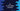# C++ if-else if-else ladder example# Introduction :

An if-else block is used to check a specific condition in C++. We can add one condition in the if block and if this condition is true, it will execute the code written inside the curly braces of the if statement. If the condition is false, it will execute the code written inside the curly braces of the else statement.

if-else ladder statement is an extended version of the simple if-else condition. It is used to check a series of expressions one by one. The first condition is checked by the if statement. If it is false, the second statement will be checked by an else if statement. We can add an infinite number of else if conditions. If all conditions are failed, else condition will be checked. else is added at the bottom of the ladder.

The syntax of if-else ladder is like below :

## Syntax of if-else ladder :

``````if(expression_1){
//code_1
}else if(expression_2){
//code_2
}else if(expression_3){
//code_3
}else{
//code_else
}
``````

You may have understood the logic of the above syntax. If the expression_1 is true, code_1 block will execute, if it is false, it will check the first else if block. The control will keep checking to the bottom until an expression results true.

else is the last expression. If none of the above condition is true, else block will run. Note that the else statement is optional.

## Example of if-else ladder in C++ :

``````#include <iostream>
using namespace std;

int main()
{
int num;
cout << "Enter a number : "; cin >> num;

if (num < 10) cout << "You have entered a number smaller than 10" << endl;
else if (num == 10) cout << "You have entered 10" << endl; else if (num > 10 && num < 20)
{
cout << "You number is within 10 and 20" << endl;
}
else if (num == 20) cout << "You have entered 20" << endl; else if (num > 20 && num < 30)
{
cout << "You number is within 20 and 30" << endl;
}
else if (num == 30) cout << "You have entered 30" << endl;
else
{
cout << "You number is greater than 30" << endl;
}

return 0;
}
``````

This is an example of if-else ladder in C++.

Here,

1. The user is asked to enter a number.

2. The number is checked if it is less than 10 or not. If yes, one message is printed.

3. Similarly, a series of else if statements are used to check that number. If a statement fails, the control moves to the next statement. Finally, else is executed if all other statements are failed.

You can see that the curly braces are optional and we can join more than two statements using a logical operator.

## Sample Example :

``````Enter a number : 10
You have entered 10

Enter a number : 3
You have entered a number smaller than 10

Enter a number : 15
You number is within 10 and 20

Enter a number : 20
You have entered 20

Enter a number : 25
You number is within 20 and 30

Enter a number : 30
You have entered 30

Enter a number : 44
You number is greater than 30
``````

## Conclusion :

In this tutorial, we have learned how to use an if-else ladder in C++. Try to run the above example and drop one comment below if you have any queries.